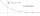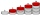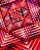# Two geometric progressions

Insert several numbers between numbers 6 and 384 so that they form with the given GP numbers and that the following applies:
a) the sum of all numbers is 510

And for another GP to apply:

b) the sum of entered numbers is -132
(These are two different geometric sequences, but with the same two members)

n =  4
q =  4
n2 =  7
q2 =  -2

### Step-by-step explanation:Did you find an error or inaccuracy? Feel free to write us. Thank you!Tips to related online calculators
Do you have a linear equation or system of equations and looking for its solution? Or do you have a quadratic equation?

## Related math problems and questions:

• Insert into GPBetween numbers 5 and 640, insert as many numbers to form geometric progression so the sum of the numbers you entered will be 630. How many numbers must you insert?
• Geometric progressionIn geometric progression, a1 = 7, q = 5. Find the condition for n to sum first n members is sn≤217.
• CrystalThe crystal grows every month 1.2 permille of its mass. For how many months to grow a crystal from weight 177 g to 384 g?
• Three members GPThe sum of three numbers in GP (geometric progression) is 21 and the sum of their squares is 189. Find the numbers.
• Sum of GP membersDetermine the sum of the GP 30, 6, 1.2, to 5 terms. What is the sum of all terms (to infinity)?
• Sequences AP + GPThe three numbers that make up the arithmetic sequence have the sum of 30. If we subtract from the first 5, from the second 4 and keep the third, we get the geometric sequence. Find AP and GP members.
• GP - three membersThe second and third of a geometric progression are 24 and 12(c+1), respectively, given that the sum of the first three terms of progression is 76. determine the value of c.
• RemaindersIt is given a set of numbers { 170; 244; 299; 333; 351; 391; 423; 644 }. Divide this numbers by number 66 and determine set of remainders. As result write sum of this remainders.
• GP membersThe geometric sequence has 10 members. The last two members are 2 and -1. Which member is -1/16?
• A bookA book contains 524 pages. If it is known that a person will select any one page between the pages numbered 125 and 384, find the probability of choosing the page numbered 252 or 253.
• SequenceBetween numbers 1 and 53 insert n members of the arithmetic sequence that its sum is 702.
• ConcertOn a Concert were sold 150 tickets for CZK 360, 235 tickets for 240 CZK and 412 for 180 CZK. How much was the total revenues for tickets?
• If the 3If the 6th term of a GP is 4 and the 10th is 4/81, find common ratio r.
• Insert 3Insert five arithmetic progression members between -7 and 3/2.
• Exponential equationFind x, if 625 ^ x = 5 The equation is exponential because the unknown is in the exponential power of 625
• Five elementThe geometric sequence is given by quotient q = 1/2 and the sum of the first six members S6 = 63. Find the fifth element a5.
• Chickens and rabbitsIn the yard were chickens and rabbits. Together they had 18 heads and 56 legs. How many chickens and how many rabbits were in the yard?